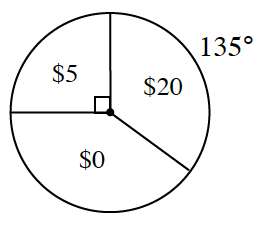### Home > INT2 > Chapter 3 > Lesson 3.1.5 > Problem3-61

3-61.

The spinner at right has three regions: $\5,\0,$ and $\20$. To play the game, you spin one time and then you win the amount in the region that the spinner lands on.

1. What is the expected value for the game?

Compare the given angle measurements to $360^\circ$.

Multiply these ratios by the amount possible to win in each section.

$\text{EV}=\5\cdot \frac{1}{4}+\0\cdot \frac{135}{360}+\20\cdot \frac{135}{360}$

2. If you have to pay $\10$ to play, is it a fair game?